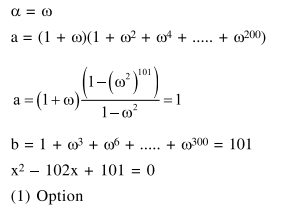# Prove the following

Question:

Let $\alpha=\frac{-1+\mathrm{i} \sqrt{3}}{2}$. If $\mathrm{a}=(1+\alpha) \sum_{\mathrm{k}=0}^{100} \alpha^{2 \mathrm{k}}$ and

$\mathrm{b}=\sum_{\mathrm{k}=0}^{100} \alpha^{3 \mathrm{k}}$, then a and $\mathrm{b}$ are the roots of the quadratic equation :

1. $x^{2}-102 x+101=0$

2. $x^{2}+101 x+100=0$

3. $x^{2}-101 x+100=0$

4. $x^{2}+102 x+101=0$

Correct Option: 1

Solution: